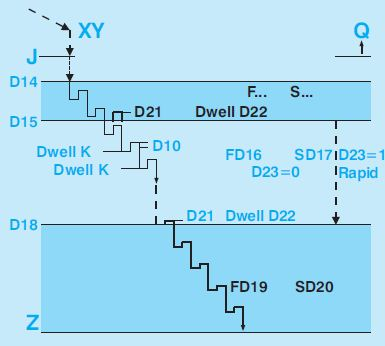# Selca G89 Differentiated drilling fixed cycle (Only for S4000 Series CNCs)

Selca G89 Differentiated drilling fixed cycle (Only for S4000 Series CNCs)

Contents

## Programming

`G89 [X... Y...] Z... J... [I...] [K...] [Q...] [F...] [S...] [D9=1] [D10=...] [D14=...] [D15=...] [D16=...] [D17=...] [D18=...] [D19=...] [D20=...] [D21=...] [D22=...] [D23=...]`## Parameters

ParameterDescription
X,Yplane hole coordinates.
Zhole bottom plane.
Jhole starting plane.
Idepth increase for chip breakage.
Qrapid return position at end of hole (Q=J if not programmed).
Kpause for chip breakage in tenths of a second (K=0 by default).
Fupper zone feed from J to D15. Last programmed F is used if in the G89 is not programmed.
Supper zone spindle speed from J to D15. Last programmed S is used if in the G89 is not programmed.
D9=1never mind where the machine is, the cycle is executed in XY position, without to program XY (D9=0 by default).
D10=rapid detachment value after each performed increase I (D10=0 by default).
D10=0 K…, no detach, K pause every I mm.
D10=… K…, D10 detach, K pause every I mm.
D10=…K0, D10 mm detach without pausing K after I mm.
D10=0 K0, equivalent to not programming I.. (cycle does not break chip).
D14=incremental position from J after which pauses are made every Imm(if I is programmed). Normally the distance between J and part surface. (D14=0 if not programmed)
D15=end of upper zone and start of intermediate zone absolute position. The zone between D15 and D18 (if D18 is programmed)or between D15 and Z is run with FD16 and SD17. The upper zone included between J and D15 is run at feed and speed programmed before G89. (D15=0 if not programmed).
D16=feed used in intermediate zone, between D15 and D18 (if D18 is programmed) or between D15 and Z (if D18 is not programmed).
D17=speed used in intermediate zone, between D15 and D18 (if D18 is programmed) or between D15 and Z (if D18 is not programmed).
D18=start of lower zone absolute position. The zone between D18 and Z is run at FD19 and SD20.
D19=feed used in lower zone between D18 and Z.
D20=speed used in lower zone between D18 and Z.
D21=detachment value in D15 and D18 positions for speed change (D21=1 if not programmed).
D22=pause after D21 detach for spindle to speed up. D21=10 (1 sec) if not programmed. Detach and pause are skipped if only feed (not speed) is changed.
D23=intermediate zone mode from D15 to D18 (D23=0 by default).
D23=0 I increases, D10 detaches and possible K
D23=1 middle zone run in rapid mode R.

Enabled: until a G80 is programmed.